# First Tutorial: Learn how to use the CTQMC impurity solver

If your DFT+EDMFTF code is already compiled, you can just take the executable ctqmc from EDMFTF/src/impurity/ctqmc/ directory (or from your bin directory), and proceed with the tutorial below.

If you did not compile the entire package, you can navigate to src/impurity/ctqmc directory, and just type make inside this directory, in order to compile ctqmc only. You will need only C++ & gsl libraries.

However, to compile you will need to edit a bit src/Makefile.in file, which is normally generated by python setup.py script during normal installation of the EDMFTF package. You will need to set PC++, PFLAGS, PLIBS. For example, for MAC computer one would set:
PC++ = mpicxx
PFLAGS = -D_MPI -O3
PLIBS = -framework Accelerate -lgsl -lgslcblas

To test the CTQMC solver, create a new directory and copy executable ctqmc, as well as python script iterate.py to this directory. Then execute it
python iterate.py
Change Coulomb repulsion "Uc" in iterate.py and reproduce the phase diagram of the Hubbard model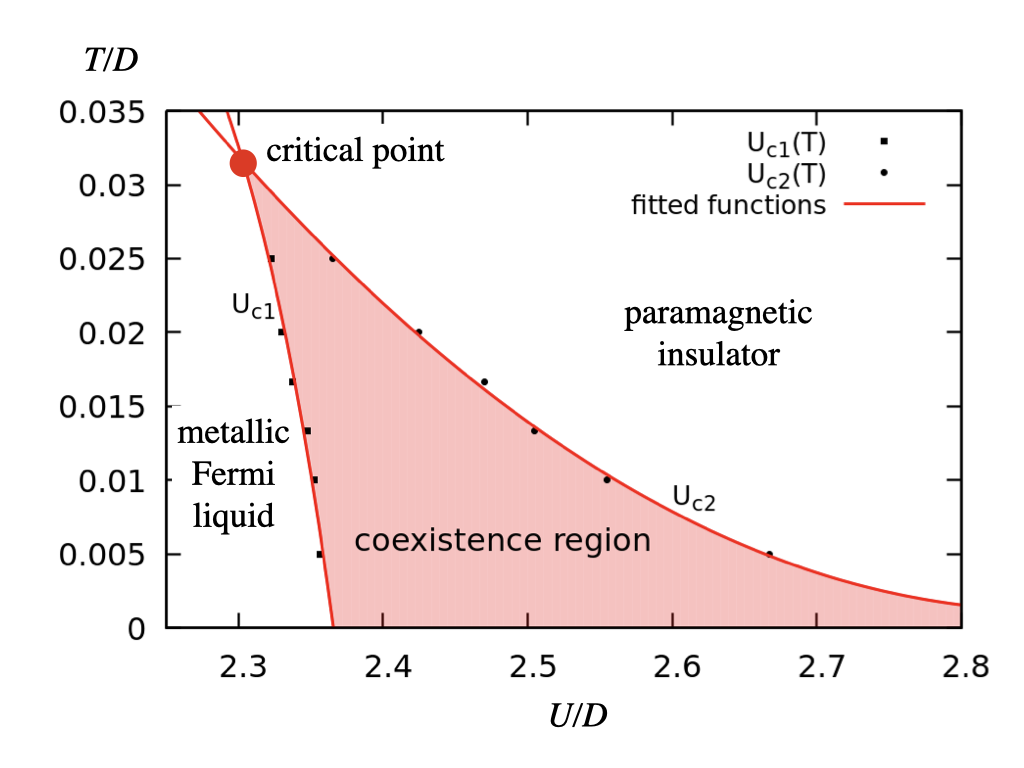First we will test the impurity solver in the single band Hubbard model. The DMFT self-consistency equation for the Bethe lattice takes the formin units of half-bandwidth D=1. To derive this equation, we notice that the impurity Green's function and the local Green's function have to coincide, which requireswhere we took into account that the impurity self-energy is equal to the local self-energy in the lattice problem. The Bethe lattice has semicircular density of states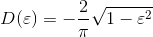which gives the following Hilbert transform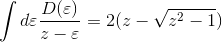and consequently, the DMFT self-consistency condition becomeswith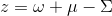This can also be cast into the formWe will thus compute the Green's function of the impurity using CTQMC solver, given a trial input hybridization function. In the next iteration we will take the resulting Green's function, multiply it by 1/4 and take it as the input hybridization. This is what the script iterate.py implements. Below we explain the parameters that should be specified to run the CTQMC impurity solver. We also list all other parmameters, which are more rarely changed, but might be useful under some circumstances.

# Details of the CTQMC solver

Most important parameters of the CTQMC solver, which are set inside iterate.py script, and are given to CTQMC solver through PARAMS file, are:

U  2.25             # Coulomb repulsion (F0)
mu 1.125            # Chemical potential
beta 100            # Inverse temperature
mode SM             # S stands for self-energy sampling, M stands for high frequency moment tail
M 5000000           # Number of Monte Carlo steps
svd_lmax 30         # number of SVD functions to project the solution
nom 80              # number of Matsubara frequency points to sample
tsample 200         # how often to record the measurements
aom 1               # number of frequency points to determin high frequency tail
GlobalFlip 1000000  # how often to perform global flip
Delta Delta.inp     # Input bath function hybridization
cix one_band.imp    # Input file with atomic state

These parameters are copied into PARAMS file, which is read by ctqmc.

Below we explain the meaning of these parameters in detail.
• U : stands for Slater F0 part of the Coulomb repulsion (note that Slater F2, F4 etc can not be changed through this PARAMS file, because they modify the atomic eigenstates in a non-trivial way, hence they have to be set inside cix file. More on that below).

• The chemical potential mu is directly related to impurity levels (more precisely to -Eimp) and, just like the Coulomb repulsion above, contains only minus the average impurity energy. This is because the crystal field splittings must be considered when the atom is diagonalized, and hence need to be present in the cix file.

• beta is the inverse temperature

•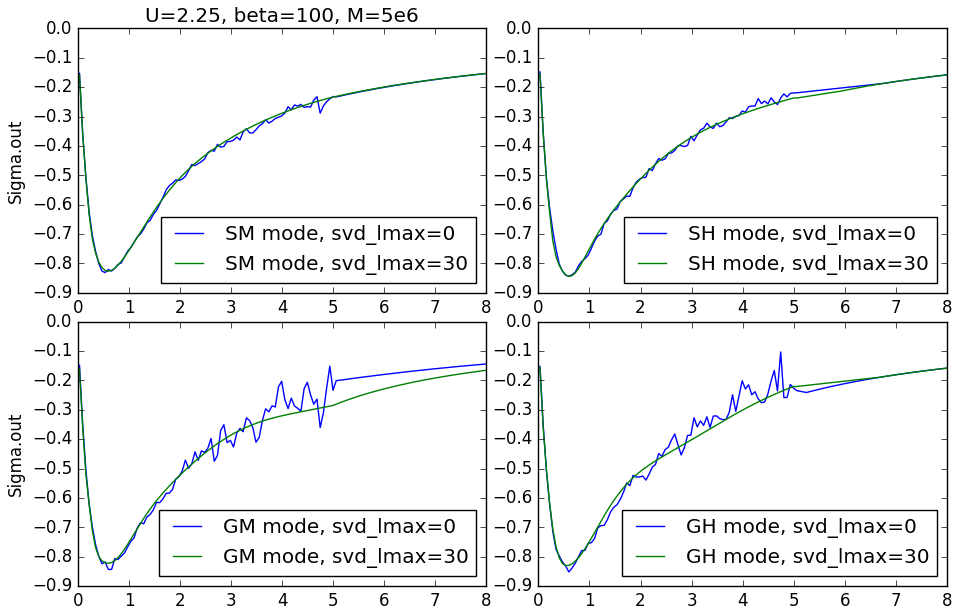• mode was introduced in March 2017 version, and can have the following values
• GM
The first letter G forces ctqmc to sample the Green's function, and than computes the self-energy through the Dyson equation (this is the conventional way). The second letter regulates the algorithm for computing the high-frequency tail of the self-energy. If the second letter is M, the high-frequency moments are calculated from the analytic expression (note that the entire four dimensional tensor of the Coulomb repulsion has to be given in the cix file). The derivation of these moments for a general case is available in High Frequency Moments. Note that the Hubbard I approximation, which is the alternative (letter H), has exactly the same first two moments of the self-energy as this analytical formula. It is safe to use H and in most cases it should not make any difference between H or M (for the second letter).
• SM
The letter S stands for sampling the self-energy directly, rather than computing it from the Green's function (using Schwinger equation). It is computed from the two-particle Green's function, as explained in the document on the two particle Green's function. This mode is available for both Ising and Full coulomb repulsion. The new version of 2018 is working in both Ising and Full case, and is now the recommended setting.
• SH
The self-energy is directly sampled, but the high frequency self-energy is computed from the Hubbard-I approximation. Of course the probabilities of the Hubbard-I self-energy are being sampled by the CTQMC. Should be almost identical to SM.
• GH
The Green's function is being sampled, and the tail is computed by Hubbard-I approximation.
Currently we recommend SH (or SM) mode (because it substantiall reduces Monte Carlo noise). Some experimenting is recommended with GH mode, as historically GH mode is most tested, and others are newer. Also note that H mode is working for a general cluster model, while S might need more tricks with the definition of U in cluster case.

• M counts the total number of Monte Carlo steps per processor. Note that in the parallel runs, each core would do M steps, and hence the run will not be faster, but it will have much better statistics than a single core run.

• svd_lmax was introduced in Mar 2017 version. If it is not set, CTQMC will sample directly in Matsubara space, resulting in more noise at high frequency, as demonstrated in the figure above. However, with svd_lmax being finite, the sampled quantity are expanded in the basis functions, which are obtained by the singular value decomposition of the kernel for analytic continuation. More on this is available in Hiroshi's paper. We recommend a value between 20-30, as 20-30 basis functions can describe any imaginary axis quantity with precision 10^-6-10^-9. However, in this mode one needs to increase t_sample (see below).

• nom stands for the number of Matsubara frequencies, which are directly sampled. When svd_lmax is not set, we directly sample nom Matsubara frequencies. When svd_lmax is nonzero, however, we could compute all Matsubara frequencies from the imaginary time SVD expansion. And such expanded self-energy and the Green's function are indeed available in Sw.dat and Gw.dat output files. However, since the high-frequency tail has to be very stable for precise total energies, we replace the high frequency part with much more precisely computed high frequency expansion, which is numerically extremely stable. This is because the Probabilities for atomic states are computed to very high precision by analytic integral over all times, hence all high frequency expansions are very precise.

• tsample : how many MC steps are taken between measurements. When svd_lmax is not set, the computation of the Green's function is linear in the number of kinks, and this parameter can be small. But with svd_lmax finite, the Green's function (or the self-energy) calculation is quadratic in the number of kinks, hence it can happen that we spend most of the time measuring, rather than moving in the phase space. It is advisable to monitor nohup_imp.xxx files (or when a single core is used it is printed on the standard output). We want to make sure that t_measure is not much larger than t_accept or t_add or t_rm.
Namely these timings shows how much time is spend in various parts of the algorithm. t_add measures the time spend in adding kinks, t_rm in removing kinks, t_mv in moving or exchanging kinks (starting in 2015 version the highly efficient exchange move is available in Ising mode, but in the March 2017 version, the same move is available also for the general Full interaction. This considerably reduces the autocorrelation time. This step is part of the t_m measurements). Further t_accept shows how much time is spend once any of these steps are accepted (hence this time is counted also in t_add, ...), and t_trial1, t_trial2, t_trial3 are times spend in trying MC moves in three stages. Finally t_measure shows how much time is spend in measuring the physical observables.
In addition to timings, the ctqmc.log file contains information on the acceptance ratio, i.e.,
Acceptance ratio: add/rm=0.2335  mv=0.545 number of accepted steps=1213234

which means that adding or removing kinks had 23% success rate and moving kinks 54% success. If these numbers are high, it is advisable to have smaller t_sample.

• aom parameter is important for sampling in Matsubara space (when svd_lmax is not set). The high frequency part can be very noisy when sampling Matsubara frequencies, and in order to add the high frequency tail in continuous way, we need to determine the value of the self-energy at the last sampled Matsubara frequency. To do that, we average over the last aom points, and than force the tail to continuously merge with the sampled data. Clearly, with projection to SVD functions (svd_lmax finite) the sampled data is much less noisy, and aom=1 is the best choice.

• GlobalFlip : how often all spin-up are exchanged with spin-down's. While other types of Global Flips (between orbitals) are available, we do not support them anymore, because they tend to be very rarely accepted.

• Delta : the name of the input hybridization function.

• cix : the name of the input cix file, which enumerates atomic/cluster eigenstates, and the matrix elements of creation operators.

There are many other parameters that could be changed through the PARAMS file, and we will summarize them below. But before we want to explain the content of cix-file.

This is the file that enumerates all atomic/cluster states, in the absence of the fermionic bath, and also contains the matrix elements of the creation operators. Hence, one needs to perform an exact diagonalization of the atom to run the ctqmc. Note that this can be very useful, as the same code can run not just the Hubbard type models, but also Kondo type models, without any modification.

We first show the cix file for the single band model, which is already included in the iterate.py script:

# cluster_size, number of states, number of baths, maximum matrix size
1 4 2 1
# baths, dimension, symmetry, global flip
0       1 0 0
1       1 0 0
# cluster energies for unique baths, eps[k]
0 0
#   N   K   Sz size F^{+,dn}, F^{+,up}, Ea  S
1   0   0    0   1   2         3        0   0
2   1   0 -0.5   1   0         4        0   0.5
3   1   0  0.5   1   4         0        0   0.5
4   2   0    0   1   0         0        0   0
# matrix elements
1  2  1  1    1    # start-state,end-state, dim1, dim2, <2|F^{+,dn}|1>
1  3  1  1    1    # start-state,end-state, dim1, dim2, <3|F^{+,up}|1>
2  0  0  0
2  4  1  1   -1    # start-state,end-state, dim1, dim2, <4|F^{+,up}|2>
3  4  1  1    1
3  0  0  0
4  0  0  0
4  0  0  0
HB2                # Hubbard-I is used to determine high-frequency
# UCoulomb : (m1,s1) (m2,s2) (m3,s2) (m4,s1)  Uc[m1,m2,m3,m4]
0 0 0 0 0.0
# number of operators needed
0


Comment lines are ignored by the code, but the code expects some string or empty line. The second line contains the cluster size (1 for single site), the number of all atomic states (which is 4 for a single impurity, i.e., empty site, spin down, spin up, and doubly occupied site). This is followed by the number of bath blocks, i.e., the type of bands that hybridize with the impurity. Here we have spin-up and spin-down bath, hence number of baths is 2. Finally, the second line specifies the maximum size of the matrix, which defines the electron creation operator. In general, it is larger than one, when we have full Coulomb interaction.

Following the third line, we have several lines, which specify the type of baths we have. Since we specified that there are two baths, we need to have here two lines, one for spin-up and one for spin-down. The first number in the line is just increasing integer, the second specifies the dimension of the bath block. Each block is one dimensional here. Next we specify which blocks are equivalent due to symmetry. Here we have paramagnetic state, hence the two baths have the same index. Finally, we have a number, which specifies which baths should be flipped by the global flip. Two baths, which have the same index, will be exchanged.

After a comment line, we have single line for each atomic state (more precisely block of atomic states). Since we have four atomic states, there are four lines. First number stands for the index, second for site occupancy, third for momentum (which is irrelevant here), then it is Sz of the atomic state. This is followed by the dimension of the block. After that, we have so-called index table.

The idea of the cix file is that we block together all atomic eigenstates, which are coupled by creation operators (this is explained in PRB 75:155113 (2007) ). Once we have all blocks enumerates, we can have an index table, which says that creation operator for spin-down changes atomic block state 1 to state state 2, and creation operator for spin-up changes atomic state 1 to state 3. We also see that atomic state 2, under the action of creation operator for up-spin, results in doubly-occupied site (state 4), and that applying creation operator for down-spin is not allowed (Pauli principle). Hence, these two columns (in general there are as many columns as there are creation operators in the problem) specify the index table.

Finally, there are two more numbers sets in each line. The first stand for the atomic energy, and the second for the total spin quantum number. Here the energy excludes the trivial term due to Coulomb repulsion F0 and the chemical potential shift mu. Inside ctqmc code, each atomic state energy will be added a term U*N*(N-1)/2.-mu*N, where U and mu are specified in the params file, and N is specified in the second column.

Now that we specified the index table for creation operator, we need to specify the matrix elements of the creation operators. The following eight lines specify such matrix elements. In general there are number-of-atomic-state*number-of-baths lines.

For each atomic state, there are two lines, because we have two baths. The first is for spin-down and the second for spin-up creation operator. Here we need to follow the order chosen above in the index table. The first number in each line stands for the state on which creation operator acts, and the second is the resulting state number. These numbers should match the index table. The next two numbers specify the dimension of the starting and the resulting block (here everything is one dimensional). For resulting states, which are not allowed by the Pauli principle, we just write 0 for dimension. Finally, we give the matrix element value. In general we would have here a matrix of dim1*dim2, where dim1 is the dimension of the starting state, and dim2 the resulting state.

The next few lines specify the high frequency tails. In this version of the code, the key word HB1 or HB2 must follow the matrix elements. This forces ctqmc to either use Hubbard-1 (HB1) or computes moments analytically (HB2). But which one is chosen, can now be specified through mode=M or H in the PARAMS file, which overwrites this settings.

If we want to calculate moments analytically, i.e., mode=M, we need to specify the tensor of Coulomb repulsion. We need to specify here only the non-zero values of the Coulomb repulsion. Each line means one non-zero value for the four dimensional tensor of the Coulomb repulsion. However, we choose to exclude the trivial F0 term, which can be added later inside ctqmc code. Hence, for the single orbital model, there is no Coulomb U beyond F0, hence we do not need to specify anything. We choose to add a single line of zeros. In general, we would have four integer numbers for the index of for orbitals, and one floating point number for the value of the Coulomb repulsion.

Finally, we can sample any number of equal time operators during the run. The matrix elements of such operators would need to be specified here. We decided not to sample any other operator.

Since the single-orbital model is perhaps to trivial, we will explain also two other more complex examples. First, the two band model, and next a plaquette with four sites, and a single orbital per site. Let's start with the two band model, which can be downloaded here:

# Cix file for cluster DMFT with CTQMC
# cluster_size, number of states, number of baths
1 16 4 1
# baths, dimension, symmetry, global-flip
0  1   0   0
1  1   0   0
2  1   1   1
3  1   1   1
# cluster energies for unique baths, eps[k]
0 0
#   N   K   Sz size
1   0   0    0  1     2   5   3   4      0   0   # 00
2   1   0  0.5  1     0   6   7   8      0   0   # u0
3   1   0  0.5  1     7  10   0   9      0   0   # 0u
4   1   0 -0.5  1     8  11   9   0      0   0   # 0d
5   1   0 -0.5  1     6   0  10  11      0   0   # d0
6   2   0    0  1     0   0  12  13      0   0   # 20
7   2   0    1  1     0  12   0  14      0   0   # uu
8   2   0    0  1     0  13  14   0      0   0   # ud
9   2   0    0  1    14  15   0   0      0   0   # 02
10  2   0    0  1    12   0   0  15      0   0   # du
11  2   0   -1  1    13   0  15   0      0   0   # dd
12  3   0  0.5  1     0   0   0  16      0   0   # 2u
13  3   0 -0.5  1     0   0  16   0      0   0   # 2d
14  3   0  0.5  1     0  16   0   0      0   0   # u2
15  3   0 -0.5  1    16   0   0   0      0   0   # d2
16  4   0    0  1     0   0   0   0      0   0   # 22
# matrix elements
1   2    1 1       1
1   5    1 1       1
1   3    1 1       1
1   4    1 1       1
2   0    0 0
.....
.....
HB2
# UCoulomb : (m1,s1) (m2,s2) (m3,s2) (m4,s1)  Uc[m1,m2,m3,m4]
0  0  0  0    0
# number of operators needed
0

Now we have 16 atomic states, and four different baths, and stil a single site.

The four baths are all written in terms of a one dimensional blocks, and we assume that there is spin degeneracy, but the self-energy for the first orbital is different than for the second (symmetry index is different for the first two and the second two baths). Global flip exchanges the first two baths, and also the second two baths, since they have the same index.

We choose no crystal field splittings between the two baths, hence eps[k] is set to zero.

Next we enumerate all states, and we give N, Sz, index table... for each state. After the comment, we also explain what each state stands for, for example up means the first orbital contains spin-up, and the second contains spin-down electron.

There are 16*4 lines of matrix elements, which we did not fully write out. One can examine them in the attached file.

Finally, let us give example of a plaquette (four-site) DMFT problem, with single orbital per-site. Here we need to perform the exact diagonalization with a chosen U=F0, because the cluster eigenvectors depend on the value of U, and the atomic state energies non-trivially depend on U. Hence, in the PARAMS file we will set U=0, and we will include U in the cix-file. We give here example for U=6t, which is close to the Mott transition.


# Cix file for cluster DMFT with CTQMC
# cluster_size, number of states, number of baths, maximum_matrix_size
4 84 8 12
# baths, dimension, symmetry, global flip
0   1 0    0   # Sz=dn, K=(0,0)
1   1 0    0   # Sz=up, K=(0,0)
2   1 1    1   # Sz=dn, K=(pi,0)
3   1 1    1   # Sz=up, K=(pi,0)
4   1 1    2   # Sz=dn, K=(0,pi)
5   1 1    2   # Sz=up, K=(0,pi)
6   1 2    3   # Sz=dn, K=(pi,pi)
7   1 2    3   # Sz=up, K=(pi,pi)
# cluster energies for non-equivalent baths, eps[k]
-2 -0 2
#   N   K   Sz size
1   0   0    0   1      2   3   4   5   6   7   8   9                  0                  0
2   1   0 -0.5   1      0  10  11  12  14  15  17  18                 -2                0.5
3   1   0  0.5   1     10   0  12  13  15  16  18  19                 -2                0.5
4   1   1 -0.5   1     11  12   0  10  17  18  14  15                  0                0.5
5   1   1  0.5   1     12  13  10   0  18  19  15  16                  0                0.5
6   1   2 -0.5   1     14  15  17  18   0  10  11  12                  0                0.5
7   1   2  0.5   1     15  16  18  19  10   0  12  13                  0                0.5
8   1   3 -0.5   1     17  18  14  15  11  12   0  10                  2                0.5
.......
# matrix elements
1   2    1   1                     1
1   3    1   1                     1
1   4    1   1                     1
1   5    1   1                     1
1   6    1   1                     1
1   7    1   1                     1
1   8    1   1                     1
1   9    1   1                     1
2   0    0   0
2  10    1   4        0.952550727929                    0       0.188982236505      -0.238606003712
2  11    1   2                     1                    0
......
HB1
# number of operators needed
0

We notice that we have now four sites, 84 blocks of atomic states (there are of course 256 eigenstates, but some of them have to be grouped into blocks, because the creation operator couples them). There are eight baths, and the maximum size of the atomic state block is 12.

Here we choose to work in the basis with momentum as a good quantum number, and we will choose baths to be enumerate by momentum and spin. We commented for each bath how was it chosen. Note that in the input/output file for ctqmc, we will find the same order of baths. But in Delta.inp we will specify only baths, which are different. Since we have only four different baths, we will give only for baths in Delta.inp file.

In the section on matrix elements, we have states of dimension higher than one. In this case, we need to specify dim1*dim2 numbers in a single row.

Finally, to perform the c-DMFT calculation for the Hubbard cluster, we give here an example of the python script iterate_cluster.py, which implements the DMFT self-consistency condition. To start, one also needs the cix-file hubbard_U_6_normal.cix. We also give the converged self-energy Sig.out, which should be the self-consistent solution at this U.

If one desires to calculate the cix file for other values of U, we want to give the tarbal with cix-file generator for the Hubbard model.

For good statistic, one should run the script on a parallel cluster with a hundred cores or so. One should specify in "mpi_prefix.dat" file, how should the parallel job be executed. For example "mpirun -n 100".

For completness we also mention more complicated example of d-wave superconducting state in plaquette DMFT.

# Cix file for cluster DMFT with CTQMC
# cluster_size, number of states, number of baths, maximum_matrix_size
4 84 6 12
# baths, dimension, symmetry, global flip
0   1       0                   0
1   1     -0*                   1
2   2       1    3    3  -1*    2
3   2       1   -3   -3  -1*    3
4   1       2                   4
5   1     -2*                   5
# cluster energies for non-equivalent baths, eps[k]
-2 -0 2  0
#   N   K   Sz size
1   0   0    0   1      3   0   5   0   7   0   9   0                  0                  0
2   1   0 -0.5   1     10   1  12   0  15   0  18   0                 -2                0.5
3   1   0  0.5   1      0   0  13   0  16   0  19   0                 -2                0.5
4   1   1 -0.5   1     12   0  10   1  18   0  15   0                  0                0.5
5   1   1  0.5   1     13   0   0   0  19   0  16   0                  0                0.5
6   1   2 -0.5   1     15   0  18   0  10   1  12   0                  0                0.5
7   1   2  0.5   1     16   0  19   0   0   0  13   0                  0                0.5
8   1   3 -0.5   1     18   0  15   0  12   0  10   1                  2                0.5

In this case, (pi,0) and (0,pi) baths mix, and become blocks of two-by-two, hence dimension of these baths is two. The conjugation and sign reversal can also be specified, as shown above. This example corresponds to the following symmetry of the Green's function: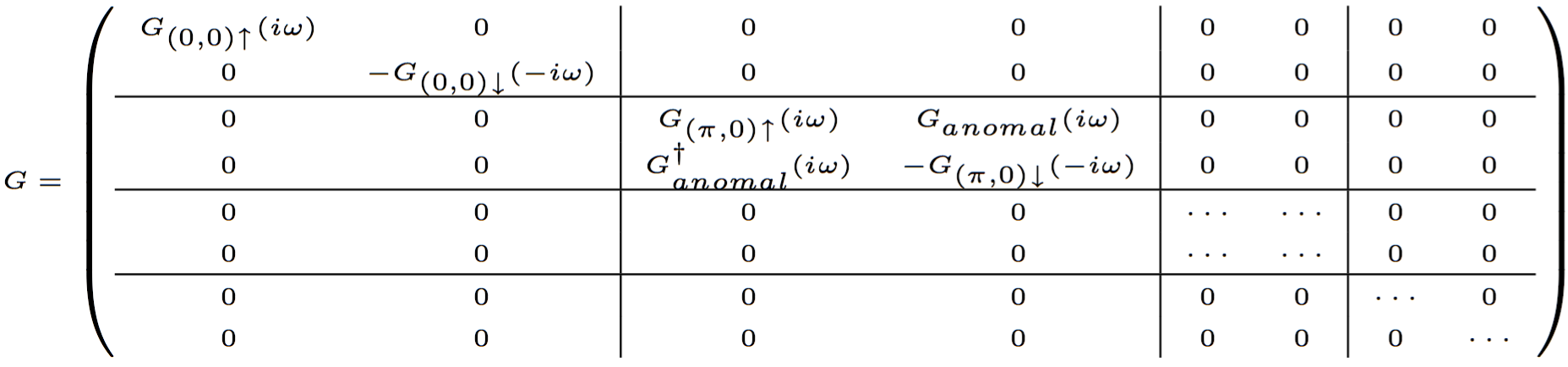Finally, as mentioned above, there are numerous other parameters, which can be changed through the PARAMS file. We list them here:
• fGf : (default "Gf.out") is the output file for green's function G(iom)
• fSig : (default "Sig.out") is the output file for self-energy Sig(iom)
• PChangeOrder : (default 0.9) The probability that we will try to add/remove a kink, rather than move/exchange kinks. This parameter needs to be increased when add/rm time (t_add, t_rm) becomes too small compared to time for moving kinks (t_mv).
• PlocalMoves (default 0.0) When using Full interaction (non-segment scheme) we can combine the local and the non-local trial moves. (In segment picture the non-local moves vanish). The non-local moves have much smaller acceptance rate, but they are much more efficient in reducing autocorrelation time (see PRB 75:155113 (2007) for explanation of global moves in general impurity problem. The local moves were introduced by Werner in the single band Hubbard model, i.e., segment picture). Since the rejections are implemented extremely efficiently (t_trial is small), the global moves are not much more expensive than the local moves, hence we use almost exclusively global moves. But one needs large number of total MC steps (larger M) to reach the same level of statistics.
• minF (default 1e-10) This parameter depends on the precision with which the matrix elements of the creation operator are computed. When we apply a creation or an annihilation operator, we check that the resulting state is not zero (excluding exponents coming from time evolution, which can be arbitrary large/small). If applying creation or annihilation operator on a state with the largest matrix element equal to unity, results in a state where all numbers are smaller than the precision with which we computed these matrix elements, than we should not accept such step. It is important to have a lower bound for that, because we can not compute this quantity to infinite precision. And if a step, which should have zero probability, is accepted because of the numerical error, it tends to ruin the entire MC run. It is mostly needed to stabilize Mott insulators.
• minM (default 1e-15) When attempting to accept a step, the absolute value of the ratio of two local traces has to be larger that minM. It is useful to have a lower bound for that, because we can not compute this quantity to infinite precision. And if a step, which should have zero probability, is accepted because of numerical error, it also tends to ruin the MC run. It is useful in Mott insulators at the boundary with the Mott transition.
• minD (default 0.0) When attempting to accept a step, the absolute value of the ratio of two determinants has to be larger than minD. Especially in Mott insulating state, it is advisable to set a lower bound between 1e-10 to 1e-15 for that. But if one is dealing with the heavy fermions, and the Kondo scale is extremely small, this parameter also needs to be very small.
• warmup (default 0) How many MC steps will be removed before we start measuring. Since DMFT is an iterative algorithm, in each successive DMFT step one has the information from the end of the previous impurity run (The entire configuration of kinks is stored in status.xxx files). The warmup is thus needed only in the first impurity calculation, and can be substantially reduced in each self-consistent successive step.
• Ntau : (default 5*beta+100) is the number of imaginary time points for interpolation of Delta(tau). We use non-linear mesh.
• Nmax : (default 50) is the maximum perturbation order in hybridization at the start. Starting in 2017 version, it will be automatically increased when needed.
• nomD : (default 0) We can combine the sampling of the self-energy and the Green's function. If nomD is finite, we take the first nomD points from the Green's function sampling (through the Dyson equation) and the rest (nom-nomD) from the self-energy sampling. This is only relevant when mode=SM or SH. Note that the self-energy is always computed also through the Dyson equation and is saved into Sig.outD file, while the sampled self-energy from the two particle Green's function is saved into Sig.outB file. These two files appear only in SM/SH mode.
• Ncout (default 500000) How often to print some basic information about the run to the nohup_imp.xxx or to the screen.
• SampleSusc (default false) By setting this parameter to 1, the spin and the charge dynamic susceptibility will be sampled and printed in susc.dat file. This can be done only when sampling directly in Matsubara space, hence svd_lmax will be switched off.
• nomb : (default 100) The number of bosonic Matsubara frequencies used to sample the spin and the charge local susceptibilities. Only relevant when SampleSusc=1.
• som (default 3) When attaching a tail to the susceptibility, we average the last som bosonic Matsubara frequency points (this is used to compute susceptibility in the same way aom is used for the self-energy).
• SampleVertex (default -1) When this is positive, it will sample the two particle Green's function and save it into tvertex.dat file. Note that when SampleVertex=1000 vertex will be measured every 1000 MC steps. The details of the two particle vertex susceptibility sampling is available in the two particle vertex document.
• nomv (default 50) The number of fermionic frequencies for computing the two particle vertex. With the combination of svd_lmax>0 this number can be arbitrary large, as high frequency values can be computed from analytic expansion. When svd_lmax=0 and vertex is sampled in Matsubara space, large nomv will slow down the sampling.
• nOm (default 1) The number of bosonic (center of mass) frequencies for vertex calculation. With the combination of svd_lmax>0 this number can be arbitrary large, while with svd_lmax=0 large nOm is expensive.
• print_vertex_xxx (default 0) If set to 1, it will print many files vertex.a.b.Om.om1, which are easy to plot and examine. They contain the same information as tvertex.dat, but here every file stands for different cuts of the two particle vertex. Here a,b are bath indices, Om is bosonic frequency, and om1 is first fermionic frequency. The second fermionic frequency is printed as the first column in each file.
• SampleTransitionP (default false) If set to 1, we will sample the transition probability, not just the probability for each atomic state.
• LazyTrace (default true) This speeds up the computation of trial probability in non-segment (Full-U) calculation. It efficiently computes trace so that most of matrix multiplications are newer performed. See (PRB 90:075149 (2014)) for details.
• saveStatus (default 1) This allows printing of status.xxx files, which remember the configuration of kinks at the end of the run.
• svd_lmax (default 0) for SVD functions, the number of SVD functions used to store G(tau) data.
• svd_L (default 10) To compute the SVD decomposition of the kernel for analytic continuation, we need to choose the cutoff on the real axis. Its default value is 10.
• svd_x0 (default 0.005) The low-energy cutoff for the same kernel. We use tangens-mesh with high-energy cutoff svd_L and low energy cutoff svd_x0.
• svd_Nw (default 500) The number of points in the real axis mesh for the kernel in SVD decomposition.
• svd_Ntau (default 5*beta+100 ) The number of points on the imaginary time when SVD decomposition of the kernel is performed.
• PMove (default 0.3) With probability (1-PChangeOrder) we will try to move a kink, or exchange two operators. With probability (1-PChangeOrder)*PMove we will move a kink, and (1-PChangeOrder)*(1-PMove) we will exchange two operators.
• BathProbability (default [1,1,1,1,...] ) This is an array of size equal to the number of baths. By default we create kinks in each bath with equal probability, and this is usually OK. However, sometimes some of the orbitals appear with very low statistics (those which are at high energy) while others (low energy orbitals) have much better statistics. You can examine the lines
acceptance add/rm for orbital =0.06132 acceptance  move  for orbital =0.06653
acceptance add/rm for orbital =0.06229 acceptance  move  for orbital =0.06582
....

in ctqmc.log, which show how different is acceptance in different orbitals. To regulate how often a kink is tried in each bath, we can set BathProbability to be different for different orbitals/baths. For example, if we have two different orbitals, and the first has much better statistics than the second, we would set BathProbability to [1,2], so that the second bath will now be tried twice as often as the first bath.
• SampleGtau (default -1) For debugging purposes, we can compute G(tau) and store it in Gtau.dat by simple binning. We can than compare it to more precise Gt.dat, which is computed by expanding G(tau) in terms of SVD basis functions. SampleGtau is counted in terms of MC steps, hence SamlpeGtau=1000 would recompute G(tau) every 1000 MC steps.
• Ncorrect (default -1) The baths with index higher than Ncorrect will not be added a high-frequency tail (if -1, all tails are added). This is sometimes useful for off-diagonal terms, which vanish, and are hard to compute from Hubbard 1 (in superconductivity, for example).
• fastFilesystem (default 0) For debugging purposes mostly. It would write out a lot more information about the run (Delta.tau current Gaver...)
• CleanUpdate (default 100000000) How often to start from scratch not relaying on accumulated information. It turns out this is not really needed, as error does not accumulate much with the MC steps.
• maxNoise (default 1e100) This is very seldon needed. Maximum amount of noise allowed in MPI_Gather Green's function. Sometimes, when statistics is bad, some processors might produce the Green's function of very bad quality. How good is the Green's function can be judged by its smoothness, and if this variation is too large, we might want to eliminate such contribution when gathering information from different cores. One needs to set maxNoise to appropriate level, like 10. This is relevant only with svd_lmax=0.
• minDeltat (default 1e-7) Delta(tau) is sometimes non-causal due to numerical error. In this case we set it to small value minDeltat.
• iseed_start (default 0) starting seed of the first core. The next core will have seed larger for 10.
• Segment (default 0) This is automatically turned to 1 when all blocks/superstates are one dimensional. When blocks are of size larger than 1, it is automatically set to zero. When segment picture is possible, this parameter can take values between 1-6. Default it is set to 1, and other schemes have slight variation in types of moves they try. They are experimental at the moment.
• Nhigh (default 40) The input hybridization function is given in Matsubara frequency, and needs to be transformed to Matsubara time. For that, we need to determine the high frequency tail, and we will determine it from the last Nhigh Matsubara points available.

## Useful output files

• nohup_imp.out.000
• appears in parallel run (the same content in the serial run is printed to the screen), and can be monitored during the run to see the progress of the code.
• Sig.out
The resulting impurity self-energy on Matsubara axis
• Gf.out
The resulting impurity Green's function on Matsubara axis
• ctqmc.log
Some log information on acceptance ratio and timings of various steps. It also contains
• mom, which specifies the density matrix,
• nf is the impurity occupancy,
• chiS0 is the spin susceptibility at zero frequency,
• chiD is the charge susceptibility at zero frequency,
• <<Sz>> is the magnetization (in units of the spin, which needs to be multiplied by 2 to get units of bohr magneton).
• Epot and Ekin stand for the potential and the kinetic energy. Notice that Epot contains not just the terms proportional to interaction U, but also the single particle terms due to crystal-field splittings. What we normally need, is the potential energy due to interaction only, which is named Tr(Sigma*G) or TrSigmaG and is mathematically equivalent to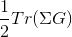• Probability.dat
• The probability for all atomic states listed in cix file. The first column stands for the index of the block of atomic states (index of the superstate), the second column is the index within the block, the third column is the probability, which should be normalized to unity. Notice that with the probability of all atomic states, and information on atomic states, one can compute many physical properties. For example, the fluctuating moment <S^2> can be computed by taking S(S+1) from each atomic state, multiplied by its probability. Similarly, the potential energy can be obtained from atomic energies and their probabilities. The density matrix can be obtained by the density matrix of each atomic state, and their probabilities.
• histogram.dat
• contains the probability for perturbation order k. Kinetic energy is <k> * T.
• susc.dat
• Contains the spin and orbital susceptibility. But is printed only when "SampleSusc=1"
• suscg.dat
• Contains the orbital susceptibility, but only when "SampleSusc=1" and the additional matrix elements are specified in cix file (see below).
• Gcoeff.dat
• The coefficient in the SVD expansion of the Green's function in imaginary time. Relevant only when svd_lmax>0.
• Gt.dat
• The imaginary time Green's function as expanded by the SVD functions. Relevant only when svd_lmax>0.
• Gtau.dat
• Appears only when SampleGtau>0. The sparsely binned Green's function, which is of lower quality than Gt.dat or Gf.out, but contains raw data simply binned.
• Gw.dat,Sw.dat
The Fourier transform of Gt.dat and the self-energy (in mode=S in which self-energy is sampled directly). Relevant only when svd_lmax>0.

## The spin, the orbital and the charge susceptibility by CTQMC

To calculate the z-component of spin-susceptibility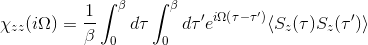or the charge susceptibilitywe use the matrix elements of Sz and N operator from cix file, and we evaluate the integral analytically for all times. To use this capability, we just need to add to PARAMS file the variable
SampleSusc  1
and the results appear in susc.dat. The first column is the spin susceptibility, and the second is the charge susceptibility.

We can also control the number of sampled bosonic frequencies by nomb parameter.

Notice that when susceptibility is sampled, we sample directly in Matsubara space and consequenctly switch svd_lmax=0.

The orbital susceptibility is a bit more challenging to sample, as it requires to apply an operator, which is not necessary commuting with the local Hamiltonian. We implemented the formula, which samples any susceptibility in the subspace spanned by the partition function. Notice that if the operator requires jumping between blocks of the local Hamiltonian (such as for example x or y component of the spin-susceptibility), it is likely nonzero in the space outside the configuration space of the partition function, and then this implementation would give wrong answer. One would need to use the worm algorithm to sample a general susceptibility. If the susceptibility corresponding to an operator O is nonzero only in the configuration space of the partition function, we can computewhere ML contains all operators in the time evolution between t=0 and t=taui-1 and MR contains operators between t=beta and t=taui. We store those time evolutions, and then we can quickly compute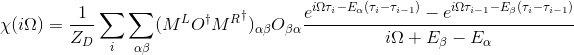To use that feature, we need to specify the matrix elements of the O operator in the cix file. After the line
# number of operators needed
we normally specify a single number
# number of operators needed
1

However, if we specify two numbers, like
# number of operators needed
1  1

it means that we will first specify a local operator, for which we require an average value <O> and after that we will specify an operator O for which we want to compute susceptibility.

The easiest way to use this feature is to run atom_d.py script, which performs exact diagonalization of the atom. We first prepare a file, which we call Trans.dat, that specifies the connection between the spherical harmonics and the orbitals used in ctqmc. Lets assume Trans.dat takes the form:

5 5 #  size of Sigind and CF
#---- Sigind follows
1    0    0    0    0
0    2    0    0    0
0    0    3    0    0
0    0    0    4    0
0    0    0    0    5
#---- CF follows
0.00000000   0.00000000    0.00000000   0.00000000    1.00000000   0.00000000    0.00000000   0.00000000    0.00000000   0.00000000
0.70710679   0.00000000    0.00000000   0.00000000    0.00000000   0.00000000    0.00000000   0.00000000    0.70710679   0.00000000
0.00000000   0.00000000    0.70710679   0.00000000    0.00000000   0.00000000   -0.70710679   0.00000000    0.00000000   0.00000000
0.00000000   0.00000000    0.00000000   0.70710679    0.00000000   0.00000000    0.00000000   0.70710679    0.00000000   0.00000000
-0.00000000  -0.70710679    0.00000000   0.00000000    0.00000000   0.00000000    0.00000000   0.00000000    0.00000000   0.70710679

In DFT+DMFT run, this information is normally stored in case.indmfl file, which you will learn in the following few tutorials. For now we just notice that Sigind is an index table for the form of the hybridization matrix, and CF is the unitary transformation from spherical to cubic harmonics. Each row stands for one cubic harmonics, and we use the order [z^2,x^2-y^2,xz,yz,xy] for the cubic harmonics, while the columns correspond to spherical harmonics with the order [-2,-1,0,1,2].

Next we can execute

atom_d.py J=0.8 l=2 OCA_G=False "CoulombF='Full'" "Eimp=[0.0,-0.1,-0.02,-0.02,-0.06]" "ORB=[0,0,1,-1,0,0,0,1,-1,0]"

which means that we choose
• Hund's coupling J=0.8eV (of the Slater form)
• l=2 means d-orbitals (five orbital model)
• OCA_G=False switches off the calculation of OCA input file
• CoulombF='Full' specifies that we will use rotationally invariant Coulomb interaction
• Eimp=[...] specifies the list of impurity levels
• Finally, ORB specifies that this additional operator for orbital susceptibility should be printed in the cix file. The list contains 10 entries, as there are 10 orbitals+spins. Note that by convention we first specify 5 orbitals of spin-down, followed by the 5 orbitals of spin-up. The operator is constructed by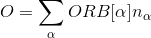For example, the specification ORB=[0,0,1,-1,0,0,0,1,-1,0] meansOnce we have such cix file with additional operator specified, we just run ctqmc with
SampleSusc  1
and the orbital susceptibility will appear in suscg.dat. The first column is the bosonic frequency, followed by the real and the imaginary part.

## Notes

Finally, some further reading on details of the CTQMC implementation is available at these lecture notes.